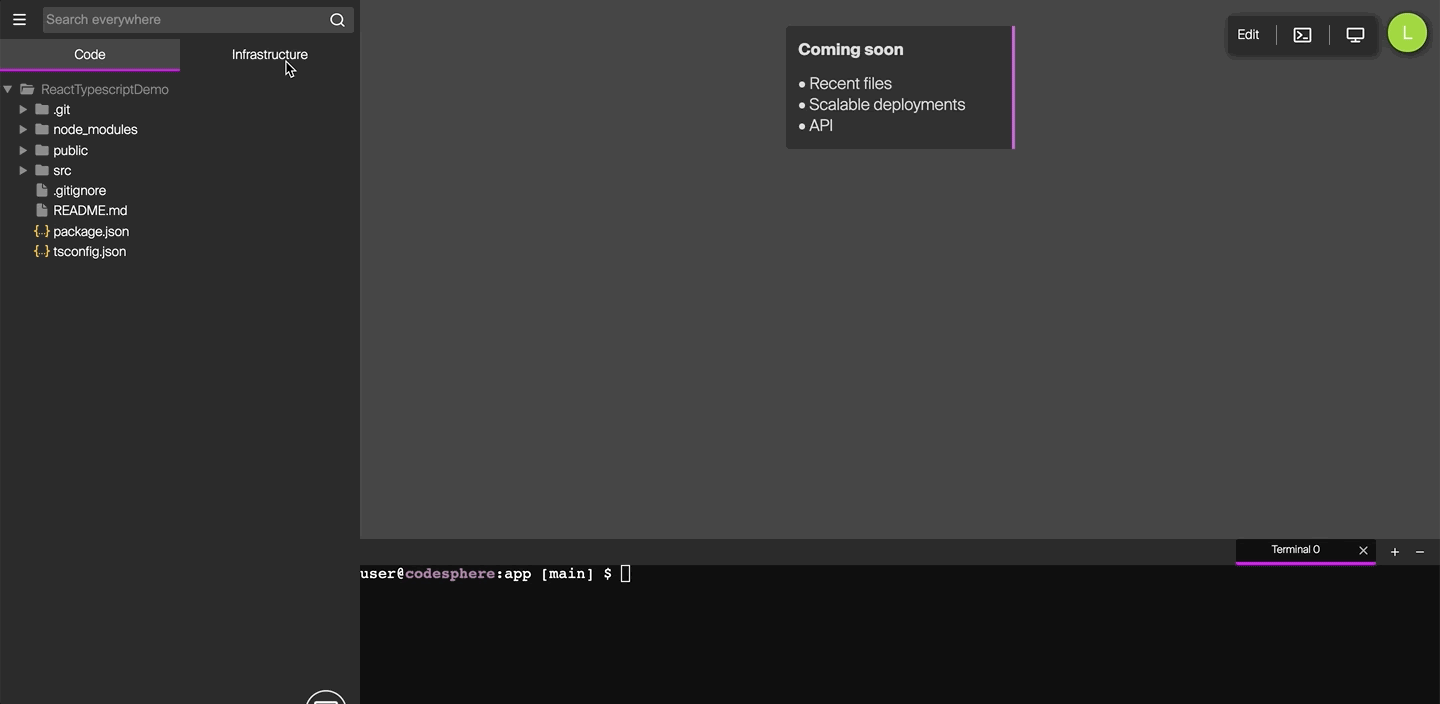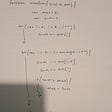# An attempt at bubble sort

I am going to attempt to explain how bubble sort works in JavaScript. The idea behind a bubble sort is to iterate through an array of integers until the integers in the array are in order. I will explain what is happening behind the scenes in a bubble sort, show my solution, and walk through the solution.

How does a bubble sort work? Let us take an array of integers consisting of 6, 2, 1, 8, 10, 9. The goal is to come back with an array with the integers in ascending order 1, 2, 6, 8, 9, 10. The way to think of this is that we need to check the first two numbers to see if the number with a lower index is greater than the number with the higher index. The array will be (arr = [2, 6, 1, 10, 8, 9])

Step 1: Is arr at index 0 greater than arr at index 1 (arr > arr). In the case below the integer at index 0 is greater than integer at index 1.

Step 2: Swap the integers so index 0 is now the lower integer (2) and index 1 is now the higher integer (6)

Step 3: We now check the next two indices in the array (index 1 and index 2) to see which is bigger.

Step 4: Integer at index 1 (6) is greater than integer at index 2 (1), so they are swapped.

Step 5: Now we check the next two indices in the array (index 2 and index 3), Is integer at index 2 (6) greater than integer at index 3 (8)? It is not so we move on to the next check.

Step 6: We check to see if the integer at index 3 (8) is greater than the integer at index 4 (10). It is not, so we move on to the next check.

Step 7: We check to see if the integer at index 4 (10) is greater than the integer at index 5 (9).

Step 8: Since the integer at index 4 (10) is greater than the integer at index 5 (9) we must swap them.

As you can see with the above image, the largest number has essentially bubbled up to the last index. We now must repeat the check for the other numbers until we iterate through the array without making a single swap. Once there are no swaps in the iteration we have completed sorting the array in ascending order.

Below is a screenshot of the algorithm I used to get this working.

Below is the screenshot of the console log of the array before the while loop (line 6) and the return array (line 19)

Let us walk through the function to see what is happening under the hood.

Lines 4 and 5 are setting up len variables as they will change during the function. Variable “len” is the length of the array, this will be getting shorter as the numbers bubble up to the right position in the function. I also have the variable “swap” set to true as this will determine if my while loop needs to continue.

Line 7 is my while loop “while (swap)”. What this is doing is it will run my while loop as long as my condition “swap” evaluates to “true”. The evaluation of this variable is determined inside of the while loop and is dependant on the for loop inside of my while loop. Remember, we are done with the bubble sort as soon as we do not make any swaps in the iteration.

Line 8 in my while loop immediately changes my “swap” variable to “false” This can change back to “true” depending on what happens in the for loop, if my for loop does not change this (meaning there was no swap) my “swap” variable evaluates to false and breaks out of my while loop.

Line 9 is the start of my for loop. I initialize an “i” variable to be “0”, my condition is as long as “i” is less than my “len” variable from line 4, and I am incrementing “i” at each loop.

Line 10 is the if statement with the logic to make the check between the indices. If the integer at index(i) is greater than the integer at index(i + 1), then run the following block of code.

Lines 11 through 14 will run only in the event that the integer at the lower index “inputArray[i]”, is greater than the integer at the higher index “inputArray[i + 1]”.

Line 11 is setting a “temp” variable to equal the higher integer, as we are resetting this integer in the next line and it is lost. On the first loop in the array, the temp will equal 6.

Line 12 is resetting the lower index in the array “inputArray[i] to be the higher integer in the check “inputArray[i+1]”. On the first loop index 0 is reset to integer 2. This will produce an array of [2, 2, 1, 10, 8, 9] as we have reset the first index to be the lower, but have not done anything with the next index “inputArray[i + 1]”

Line 13 is resetting the higher index in the check “inputArray[i + 1]” to be equal to what the variable “temp” is, as it was the higher value and we lost it once we reset the lower index “inputArray[i]”. This will produce an array of [2, 6, 1, 10, 8, 9].

Line 14 is resetting our “swap” variable to “true” as we did make a swap in the for loop and will have to continue the while loop as “swap” now evaluates to “true”.

Note: The for loop will run while the “i” variable is less than the length of “len”.

Line 17 is the last line in the while loop, this is lowering the length of variable “len” by one since there is no need to check the last value in our array as the largest number has bubbled up and it is in its right position in the array. This will repeat each time the for loop has iterated through the array.

Since “swap” is still “true” the while loop will now begin again. Once we have run through the for loop and the “if” condition in line 10 evaluates to false will line 8, “swap = false” remain, and the while loop will break. This means there were no swaps and the array is completely sorted. Keep in mind this is not the most efficient sort as you are iterating through the array multiple times. This was still a fun learning experience and while there is a lot happening here, I hope this was helpful.

--

--

--

## More from Jr Medina

Soon-to-be Flatiron School Successor, with an enthusiasm towards Front-End Web Development, lifelong learning, and continuous improvement.

Love podcasts or audiobooks? Learn on the go with our new app.

## I will do unique front end work pixel perfect## Operation & Operators > JavaScript — 2## Understanding Functional Programming with Javascript## How to Use Environment Variables in Codesphere## TypeScript Tip of the Week — Optional Chaining## QR code scanner LWC Component## Jr Medina

Soon-to-be Flatiron School Successor, with an enthusiasm towards Front-End Web Development, lifelong learning, and continuous improvement.

## Develop Your Personality With These Simple Tricks## Type. click. delete. change.## Today we talk about some of algorithms like Sliding Window technique.